# Canonical Constructive Systems Ori Lahav under the supervision

• Slides: 33Canonical Constructive Systems Ori Lahav under the supervision of Prof. Arnon Avron 28 July 2009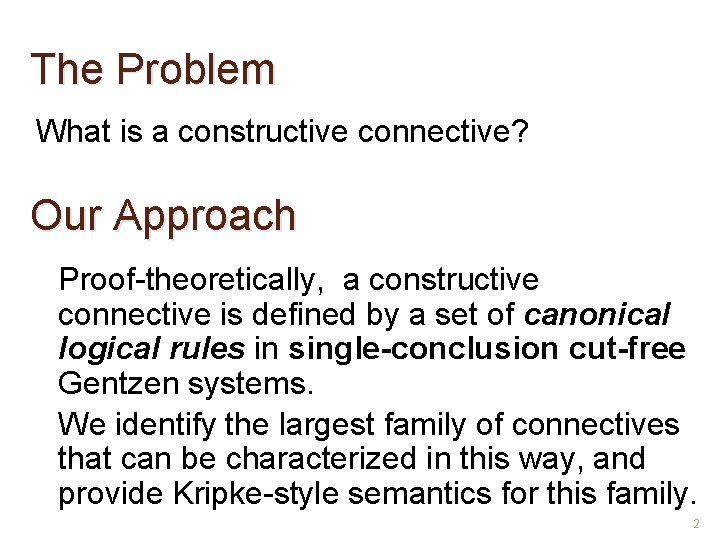The Problem What is a constructive connective? Our Approach Proof-theoretically, a constructive connective is defined by a set of canonical logical rules in single-conclusion cut-free Gentzen systems. We identify the largest family of connectives that can be characterized in this way, and provide Kripke-style semantics for this family. 2Strict vs. Non-Strict sequential system Non-strict sequential system Only definite sequents (sequents of the form ) are used in derivations. Also negative sequents (sequents of the form ) are used in derivations. � The strict version is more natural to induce an tcr. � Gentzen’s original single-conclusion sequential system (LJ) was non-strict. � Previous papers (Bowen 71, Kaminsky 88 and Ciabattoni and Terui 06) consider non-strict systems. � We begin this presentation with the strict version, and postpone the non -strict case. 3Example: Strict Canonical Rules for Implication Rule Introduction p 1 p 2 / p 1 p 2 Elimination p 1 p 2 / p 1 p 2 Application 4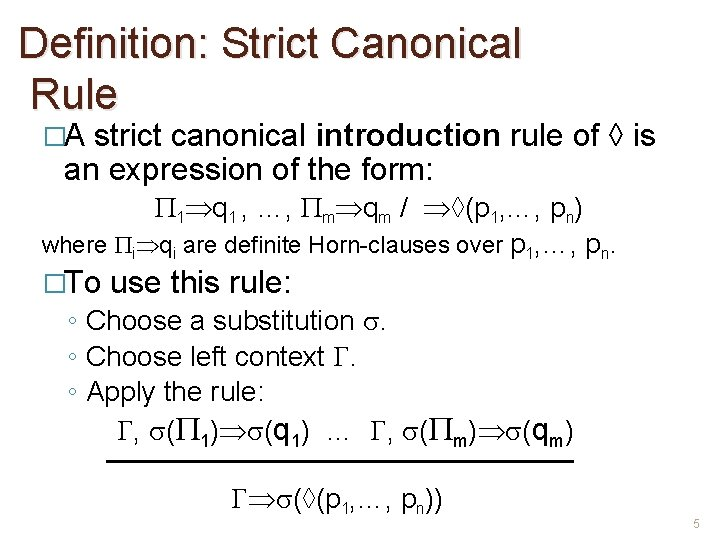Definition: Strict Canonical Rule �A strict canonical introduction rule of ◊ is an expression of the form: 1 q 1 , …, m qm / ◊(p 1, …, pn) where i qi are definite Horn-clauses over p 1, …, pn. �To use this rule: ◦ Choose a substitution . ◦ Choose left context . ◦ Apply the rule: , ( 1) (q 1) … , ( m) (qm) (◊(p 1, …, pn)) 5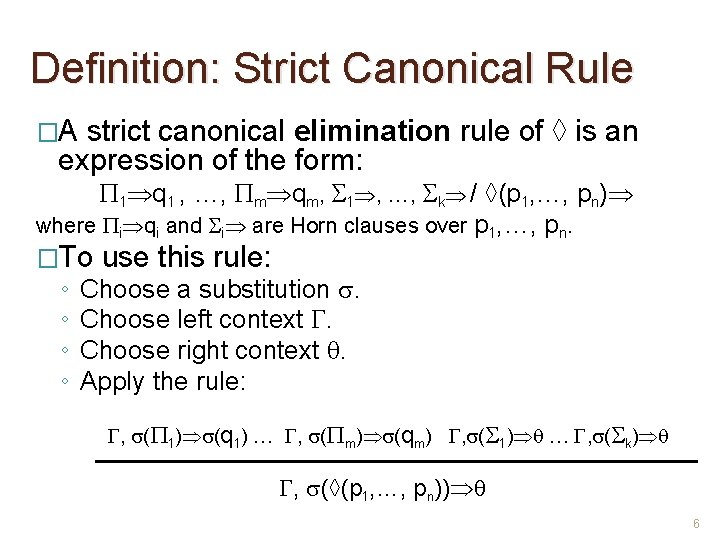Definition: Strict Canonical Rule �A strict canonical elimination rule of ◊ is an expression of the form: 1 q 1 , …, m qm, 1 , …, k / ◊(p 1, …, pn) where i qi and i are Horn clauses over p 1, …, pn. �To ◦ ◦ use this rule: Choose a substitution . Choose left context . Choose right context . Apply the rule: , ( 1) (q 1) … , ( m) (qm) , ( 1) … , ( k) , (◊(p 1, …, pn)) 6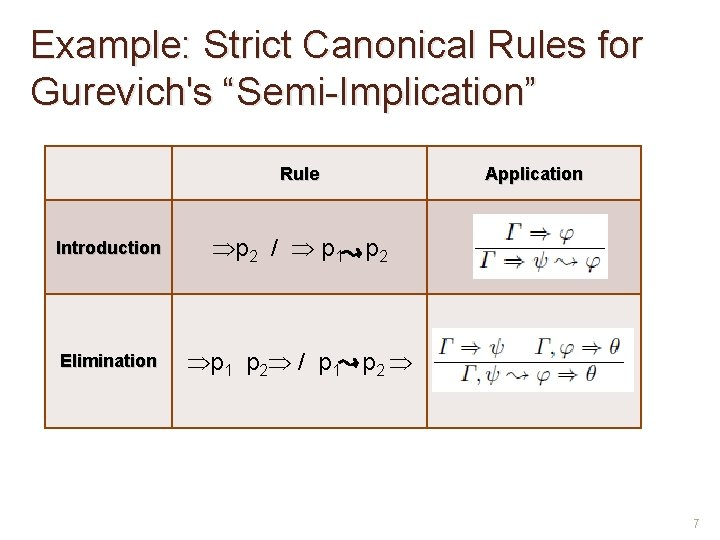Example: Strict Canonical Rules for Gurevich's “Semi-Implication” Rule Introduction p 2 / p 1 p 2 Elimination p 1 p 2 / p 1 p 2 Application 7Strict Canonical (Single-Conclusion) Systems • Axioms Structural Rules Logical Rules • Cut , , Weakening , • Strict Canonical Introduction and Elimination Rules 8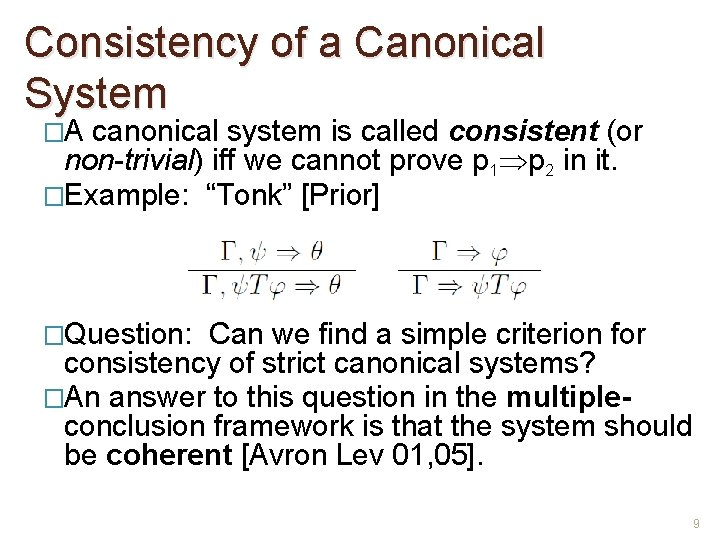Consistency of a Canonical System �A canonical system is called consistent (or non-trivial) iff we cannot prove p 1 p 2 in it. �Example: “Tonk” [Prior] �Question: Can we find a simple criterion for consistency of strict canonical systems? �An answer to this question in the multipleconclusion framework is that the system should be coherent [Avron Lev 01, 05]. 9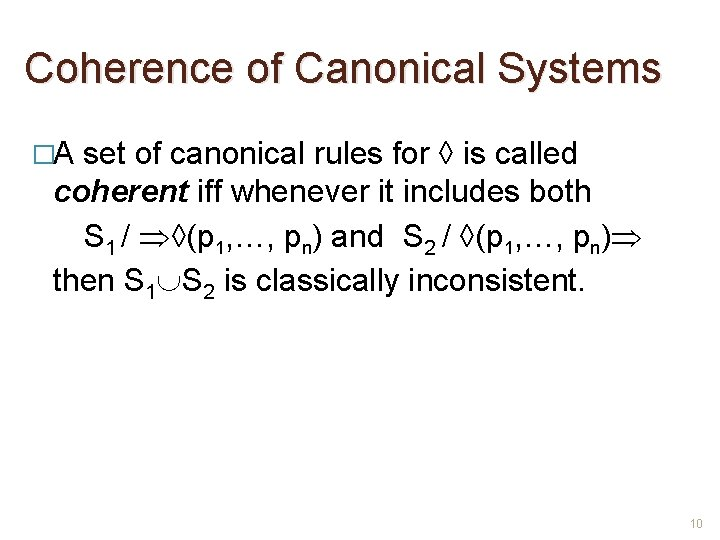Coherence of Canonical Systems �A set of canonical rules for ◊ is called coherent iff whenever it includes both S 1 / ◊(p 1, …, pn) and S 2 / ◊(p 1, …, pn) then S 1 S 2 is classically inconsistent. 10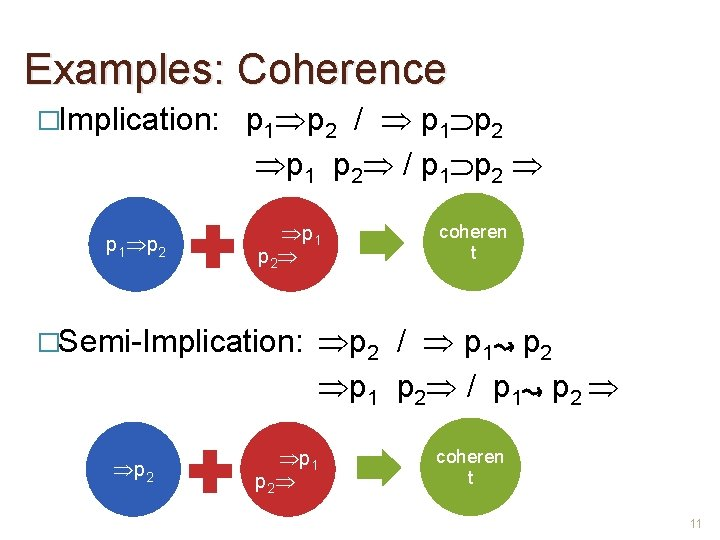Examples: Coherence �Implication: p 1 p 2 / p 1 p 2 �Semi-Implication: p 2 coheren t p 2 / p 1 p 2 / p 1 p 2 coheren t 11Examples: Coherence �“Tonk”: p 2 / p 1 T p 2 p 1 / p 1 T p 2 �This p 1 NOT coheren t is what is wrong with “Tonk”. 12Coherence and Consistency �Theorem: Coherence is necessary and sufficient for consistency of strict canonical system. �A strict canonical system is called constructive iff every connective has a coherent set of rules. 13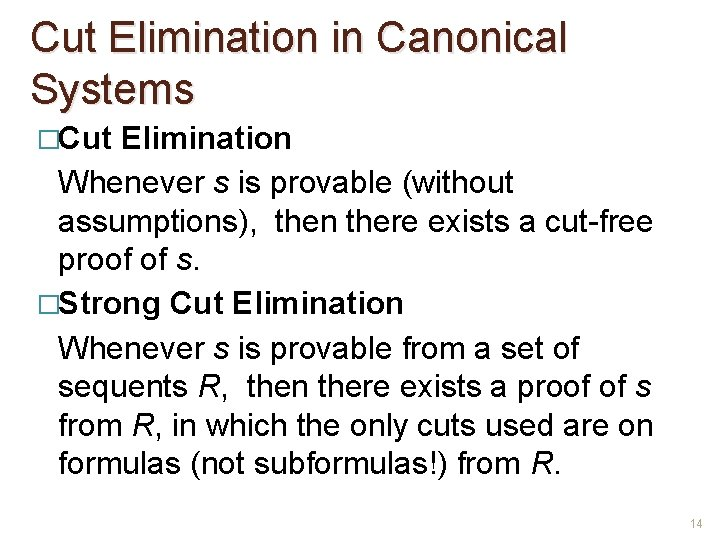Cut Elimination in Canonical Systems �Cut Elimination Whenever s is provable (without assumptions), then there exists a cut-free proof of s. �Strong Cut Elimination Whenever s is provable from a set of sequents R, then there exists a proof of s from R, in which the only cuts used are on formulas (not subformulas!) from R. 14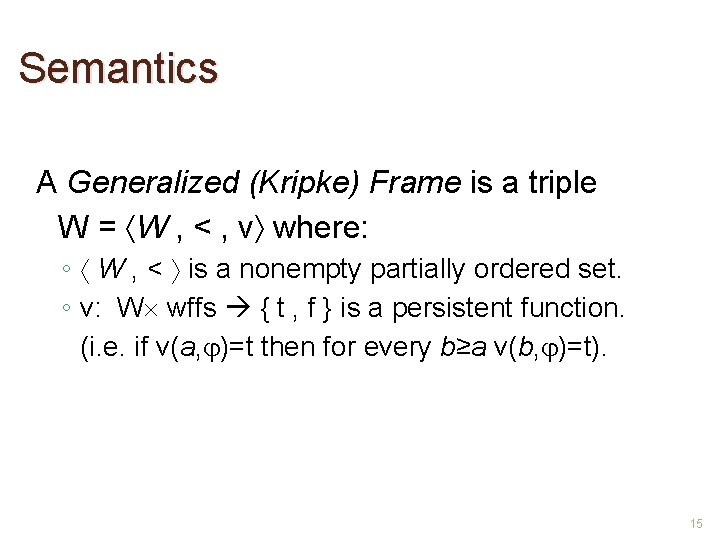Semantics A Generalized (Kripke) Frame is a triple W = W , < , v where: ◦ W , < is a nonempty partially ordered set. ◦ v: W wffs { t , f } is a persistent function. (i. e. if v(a, )=t then for every b≥a v(b, )=t). 15Semantics Let W = W , < , v be a generalized frame: ◦ A sequent is locally true in a W if either v(a, )=f for some , or v(a, )=t for some . ◦ A sequent is true in a W if it is locally true in every b ≥ a. ◦ W is a model of a sequent if it is locally true in every a W. 16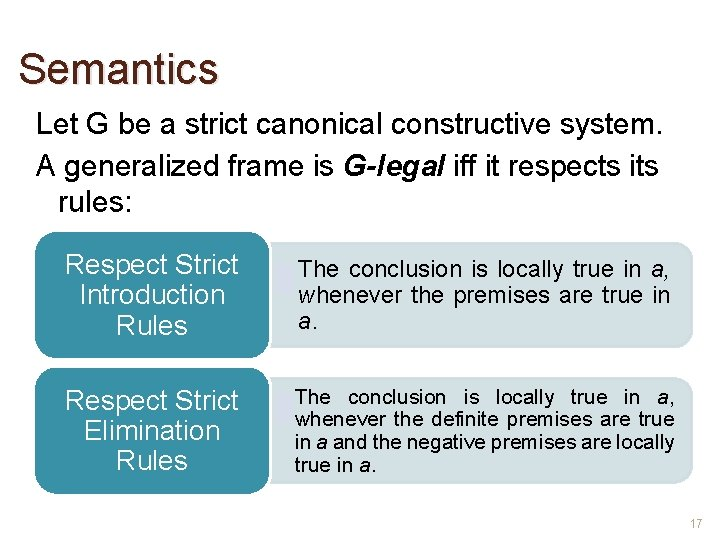Semantics Let G be a strict canonical constructive system. A generalized frame is G-legal iff it respects its rules: Respect Strict Introduction Rules • The conclusion is locally true in a, whenever the premises are true in a. Respect Strict Elimination Rules • The conclusion is locally true in a, whenever the definite premises are true in a and the negative premises are locally true in a. 17Example: Semantics of Implication p 1 p 2 / p 1 p 2 v(a, )=t if v(b, )=f or v(b, )=t for every b ≥ a v(a, )=f if v(a, )=t and v(a, )=f exactly the well-known Kripke semantics for intuitionistic implication. 18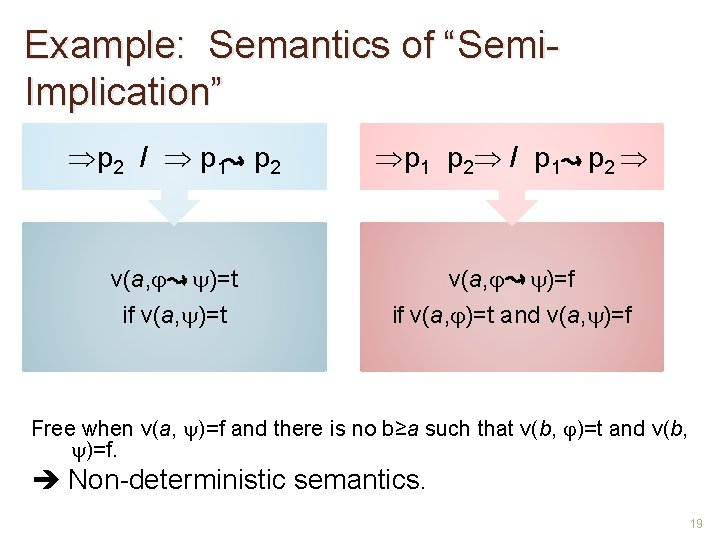Example: Semantics of “Semi. Implication” p 2 / p 1 p 2 / p 1 p 2 v(a, )=t if v(a, )=t v(a, )=f if v(a, )=t and v(a, )=f Free when v(a, )=f and there is no b≥a such that v(b, )=t and v(b, )=f. Non-deterministic semantics. 19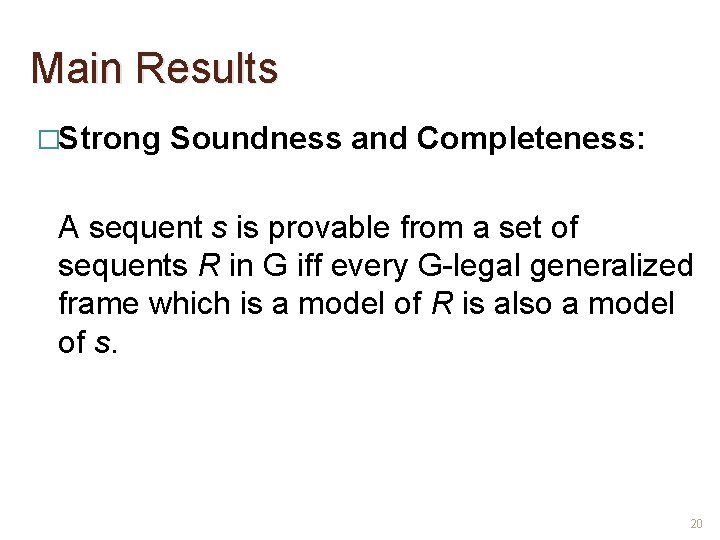Main Results �Strong Soundness and Completeness: A sequent s is provable from a set of sequents R in G iff every G-legal generalized frame which is a model of R is also a model of s. 20Main Results �General Strong Cut Elimination: Every strict canonical constructive system admits strong cut-elimination. �Decidability: Every strict canonical constructive system is decidable. �Modularity: The characterization of a constructive connective is independent of the system in which it is included. 21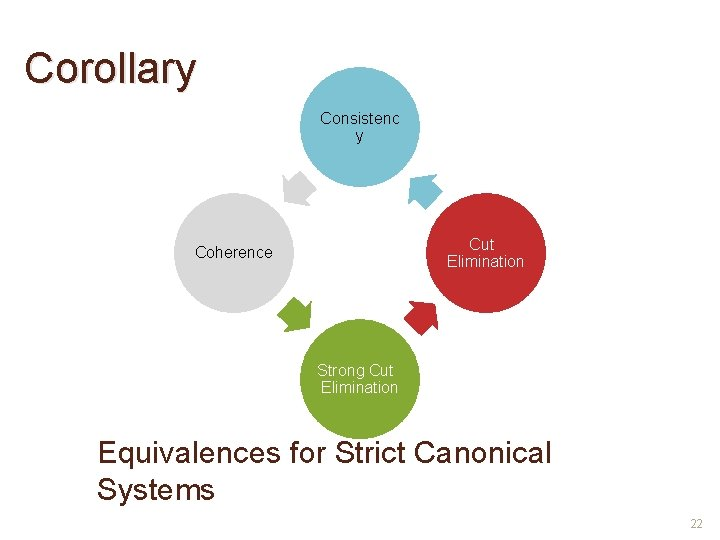Corollary Consistenc y Cut Elimination Coherence Strong Cut Elimination Equivalences for Strict Canonical Systems 22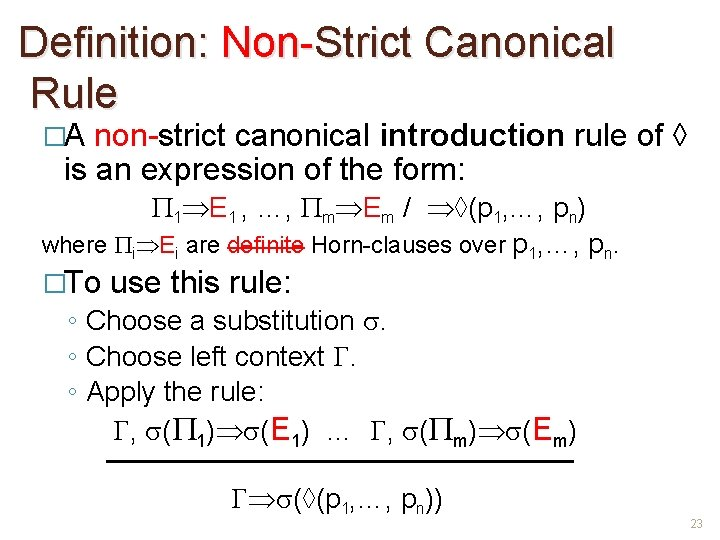Definition: Non-Strict Canonical Rule �A non-strict canonical introduction rule of ◊ is an expression of the form: 1 E 1 , …, m Em / ◊(p 1, …, pn) where i Ei are definite Horn-clauses over p 1, …, pn. �To use this rule: ◦ Choose a substitution . ◦ Choose left context . ◦ Apply the rule: , ( 1) (E 1) … , ( m) (Em) (◊(p 1, …, pn)) 23Definition: Non-Strict Canonical Rule �A non-strict canonical elimination rule of ◊ is an expression of the form: { 1 E 1 , …, m Em } , { 1 , …, k } / ◊(p 1, …, pn) where i Ei and i are Horn clauses over p 1, …, �To ◦ ◦ use this rule: p n. Choose a substitution . Choose left context . Choose right context E. Apply the rule: , ( 1) (E 1) … , ( m) (Em) , ( 1) E … , ( k) E , (◊(p 1, …, pn)) E 24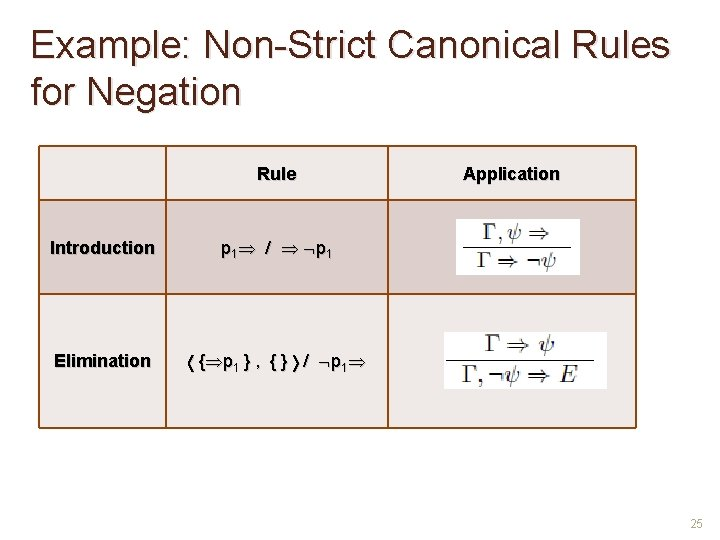Example: Non-Strict Canonical Rules for Negation Rule Introduction p 1 / p 1 Elimination { p 1 } , { } / p 1 Application 25Example: Non-Strict Canonical Rules for Bowen’s “Not-Both” Rule Introduction Elimination Application p 1 / p 1|p 2 { p 1 , p 2 } , { } / p 1|p 2 26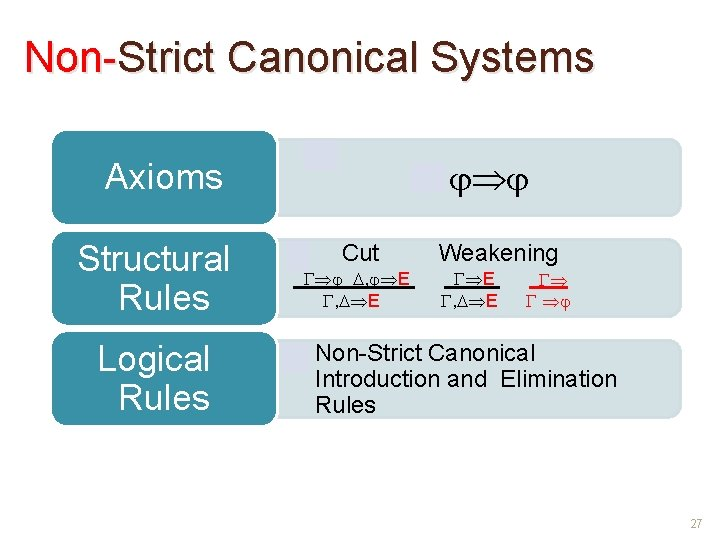Non-Strict Canonical Systems • Axioms Structural Rules Logical Rules • Cut , E Weakening E , E • Non-Strict Canonical Introduction and Elimination Rules 27Semantics Let G be a non-strict canonical constructive system. A generalized frame is G-legal iff it respects its rules: Respect Non. Strict Introduction Rules • The conclusion is locally true in a, whenever the premises are true in a. Respect Non. Strict Elimination Rules • The conclusion is locally true in a, whenever the definite hard premises are true in a and the negative soft premises are locally true in a. 28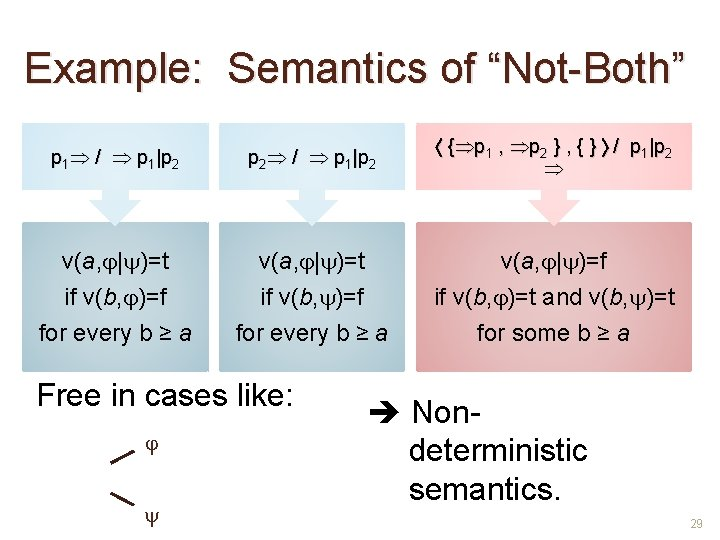Example: Semantics of “Not-Both” p 1 / p 1|p 2 { p 1 , p 2 } , { } / p 1|p 2 v(a, | )=t if v(b, )=f for every b ≥ a v(a, | )=f if v(b, )=t and v(b, )=t for some b ≥ a Free in cases like: Nondeterministic semantics. 29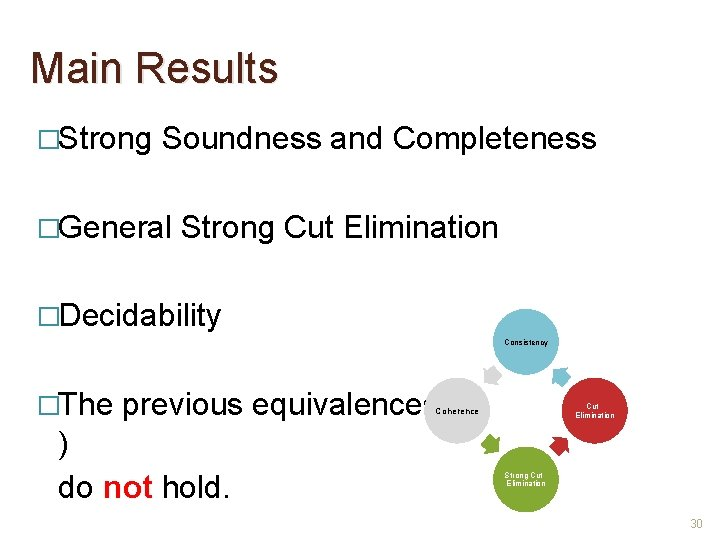Main Results �Strong Soundness and Completeness �General Strong Cut Elimination �Decidability Consistency �The previous equivalences ( ) do not hold. Cut Elimination Coherence Strong Cut Elimination 30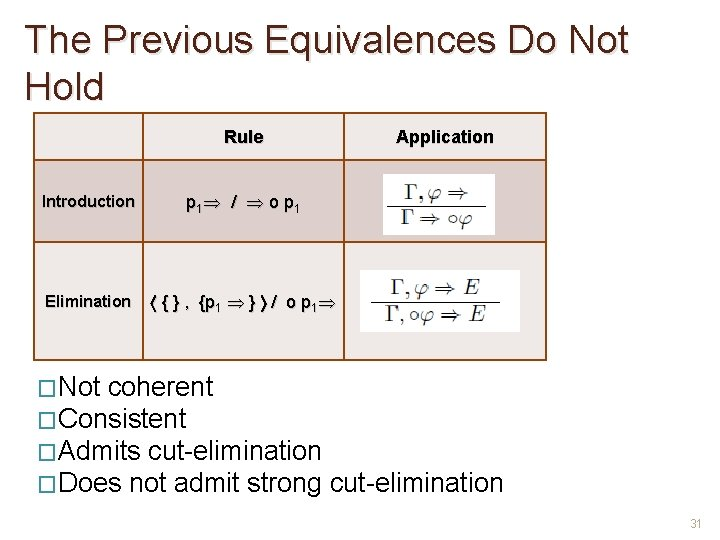The Previous Equivalences Do Not Hold Rule Application Introduction p 1 / o p 1 Elimination { } , { p 1 } / o p 1 �Not coherent �Consistent �Admits cut-elimination �Does not admit strong cut-elimination 31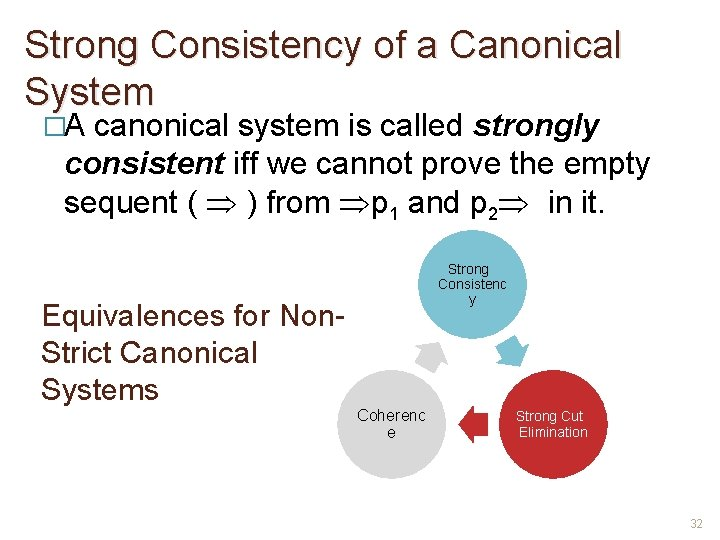Strong Consistency of a Canonical System �A canonical system is called strongly consistent iff we cannot prove the empty sequent ( ) from p 1 and p 2 in it. Equivalences for Non. Strict Canonical Systems Strong Consistenc y Coherenc e Strong Cut Elimination 32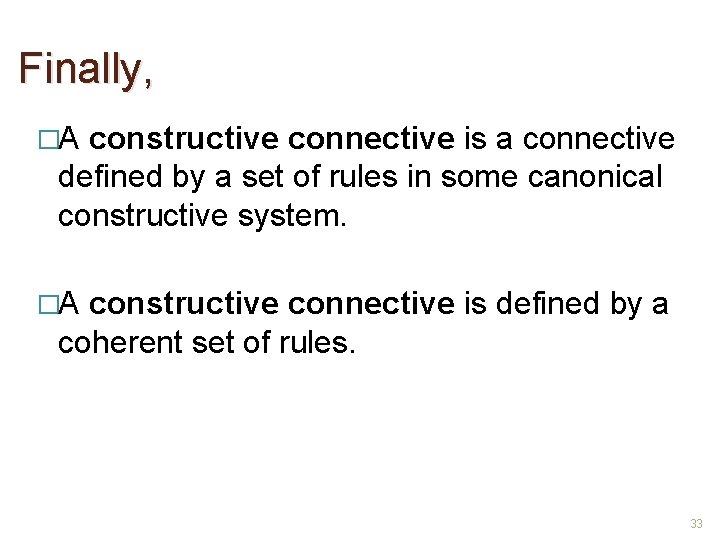Finally, �A constructive connective is a connective defined by a set of rules in some canonical constructive system. �A constructive connective is defined by a coherent set of rules. 33